• +91 9971497814
• info@interviewmaterial.com

# Chapter 15 Statistics Ex-15.1 Interview Questions Answers

### Related Subjects

Question 1 : Find the mean deviation about the mean for the data in Exercises 1 and 2.
4, 7, 8, 9, 10, 12, 13, 17

First we have to find (x̅) of the given data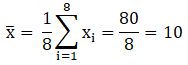So, the respective values of the deviations from mean,
i.e., xi – x̅ are, 10 – 4 = 6, 10 – 7 = 3, 10 – 8 = 2, 10 – 9 = 1, 10 – 10 = 0,
10 – 12 = – 2, 10 – 13 = – 3, 10 – 17 = – 7
6, 3, 2, 1, 0, -2, -3, -7
Now absolute values of the deviations,
6, 3, 2, 1, 0, 2, 3, 7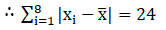MD = sum of deviations/ number of observations
= 24/8
= 3
So, the mean deviation for the given data is 3.

Question 2 : Find the mean deviation about the mean for the data in Exercises 1 and 2.
38, 70, 48, 40, 42, 55, 63, 46, 54, 44

First we have to find (x̅) of the given data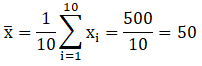So, the respective values of the deviations from mean,
i.e., xi – x̅ are, 50 – 38 = -12, 50 -70 = -20, 50 – 48 = 2, 50 – 40 = 10, 50 – 42 = 8,
50 – 55 = – 5, 50 – 63 = – 13, 50 – 46 = 4, 50 – 54 = -4, 50 – 44 = 6
-12, 20, -2, -10, -8, 5, 13, -4, 4, -6
Now absolute values of the deviations,
12, 20, 2, 10, 8, 5, 13, 4, 4, 6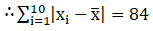MD = sum of deviations/ number of observations
= 84/10
= 8.4
So, the mean deviation for the given data is 8.4.

Question 3 : Find the mean deviation about the median for the data in Exercises 3 and 4.
13, 17, 16, 14, 11, 13, 10, 16, 11, 18, 12, 17

First we have toarrange the given observations into ascending order,

10, 11, 11, 12, 13,13, 14, 16, 16, 17, 17, 18.

The number ofobservations is 12

Then,

Median = ((12/2)th observation+ ((12/2)+ 1)th observation)/2

(12/2)th observation= 6th = 13

(12/2)+ 1)th observation= 6 + 1

= 7th =14

Median = (13 + 14)/2

= 27/2

= 13.5

So, the absolutevalues of the respective deviations from the median, i.e., |xi –M| are

3.5, 2.5, 2.5, 1.5,0.5, 0.5, 0.5, 2.5, 2.5, 3.5, 3.5, 4.5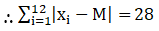Mean Deviation,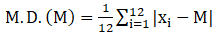= (1/12) × 28

= 2.33

So, the mean deviationabout the median for the given data is 2.33.

Question 4 : Find the mean deviation about the median for the data in Exercises 3 and 4.
36, 72, 46, 42, 60, 45, 53, 46, 51, 49

First we have toarrange the given observations into ascending order,

36, 42, 45, 46, 46,49, 51, 53, 60, 72.

The number ofobservations is 10

Then,

Median = ((10/2)th observation+ ((10/2)+ 1)th observation)/2

(10/2)th observation= 5th = 46

(10/2)+ 1)th observation= 5 + 1

= 6th =49

Median = (46 + 49)/2

= 95

= 47.5

So, the absolutevalues of the respective deviations from the median, i.e., |xi –M| are

11.5, 5.5, 2.5, 1.5,1.5, 1.5, 3.5, 5.5, 12.5, 24.5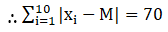Mean Deviation,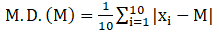= (1/10) × 70

= 7

So, the mean deviationabout the median for the given data is 7.

Question 5 : Find the mean deviation about the mean for the data in Exercises 5 and 6.
 xi 5 10 15 20 25 fi 7 4 6 3 5

Let us make the tableof the given data and append other columns after calculations.

 Xi fi fixi |xi – x̅| fi |xi – x̅| 5 7 35 9 63 10 4 40 4 16 15 6 90 1 6 20 3 60 6 18 25 5 125 11 55 25 350 158

The sum of calculateddata,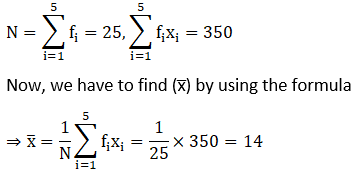The absolute values ofthe deviations from the mean, i.e., |xi – x̅|, as shown in thetable.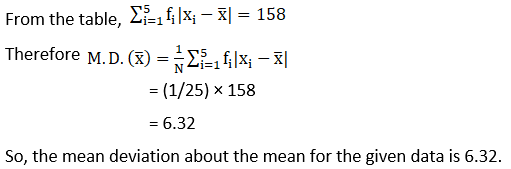Question 6 : Find the mean deviation about the mean for the data in Exercises 5 and 6.
 xi 10 30 50 70 90 fi 4 24 28 16 8

Answer 6 : Let us make the table of the given data and append other columns after calculations.

 Xi fi fixi |xi – x̅| fi |xi – x̅| 10 4 40 40 160 30 24 720 20 480 50 28 1400 0 0 70 16 1120 20 320 90 8 720 40 320 80 4000 1280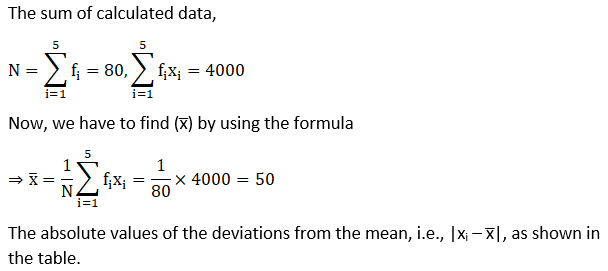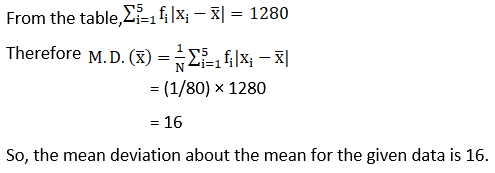Question 7 : Find the mean deviation about the median for the data in Exercises 7 and 8.
 xi 5 7 9 10 12 15 fi 8 6 2 2 2 6

Answer 7 : Let us make the table of the given data and append other columns after calculations.

 Xi fi c.f. |xi – M| fi |xi – M| 5 8 8 2 16 7 6 14 0 0 9 2 16 2 4 10 2 18 3 6 12 2 20 5 10 15 6 26 8 48

Now, N = 26, which iseven.

Median is the mean ofthe 13th and 14th observations. Both of theseobservations lie in the cumulative frequency 14, for which the correspondingobservation is 7.

Then,

Median = (13th observation+ 14th observation)/2

= (7 + 7)/2

= 14/2

= 7

So, the absolutevalues of the respective deviations from the median, i.e., |xi –M| are shown in the table.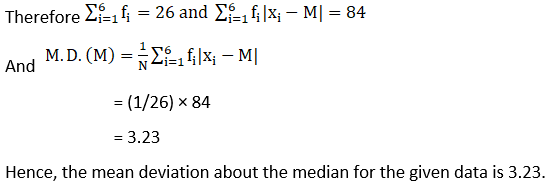Question 8 : Find the mean deviation about the median for the data in Exercises 7 and 8.
 xi 15 21 27 30 35 fi 3 5 6 7 8

Let us make the tableof the given data and append other columns after calculations.

 Xi fi c.f. |xi – M| fi |xi – M| 15 3 3 15 45 21 5 8 9 45 27 6 14 3 18 30 7 21 0 0 35 8 29 5 40

Now, N = 29, which isodd.

So 29/2 = 14.5

The cumulativefrequency greater than 14.5 is 21, for which the corresponding observation is30.

Then,

Median = (15th observation+ 16th observation)/2

= (30 + 30)/2

= 60/2

= 30

So, the absolutevalues of the respective deviations from the median, i.e., |xi –M| are shown in the table.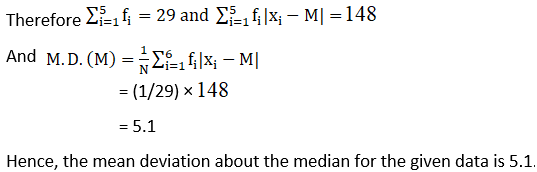Question 9 : Find the mean deviation about the mean for the data in Exercises 9 and 10.
 Income per day in ₹ 0 – 100 100 – 200 200 – 300 300 – 400 400 – 500 500 – 600 600 – 700 700 – 800 Number of persons 4 8 9 10 7 5 4 3

Let us make the tableof the given data and append other columns after calculations.

 Income per day in ₹ Number of persons fi Mid – points xi fixi |xi – x̅| fi|xi – x̅| 0 – 100 4 50 200 308 1232 100 – 200 8 150 1200 208 1664 200 – 300 9 250 2250 108 972 300 – 400 10 350 3500 8 80 400 – 500 7 450 3150 92 644 500 – 600 5 550 2750 192 960 600 – 700 4 650 2600 292 1160 700 – 800 3 750 2250 392 1176 50 17900 7896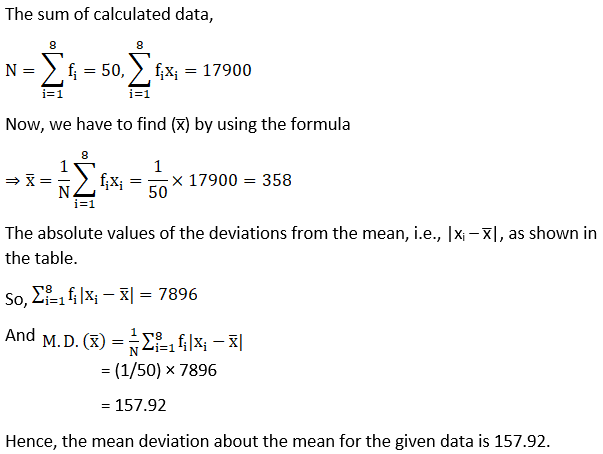Question 10 : Find the mean deviation about the mean for the data in Exercises 9 and 10.
 Height in cms 95 – 105 105 – 115 115 – 125 125 – 135 135 – 145 145 – 155 Number of boys 9 13 26 30 12 10

Let us make the tableof the given data and append other columns after calculations.

 Height in cms Number of boys fi Mid – points xi fixi |xi – x̅| fi|xi – x̅| 95 – 105 9 100 900 25.3 227.7 105 – 115 13 110 1430 15.3 198.9 115 – 125 26 120 3120 5.3 137.8 125 – 135 30 130 3900 4.7 141 135 – 145 12 140 1680 14.7 176.4 145 – 155 10 150 1500 24.7 247 100 12530 1128.8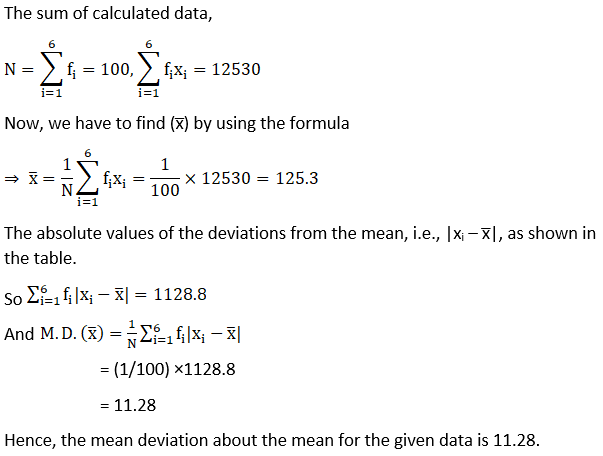Todays Deals### Chapter 15 Statistics Ex-15.1 Contributorskrishan

Name:
Email:

# Latest News# 9000 interview questions in different categories